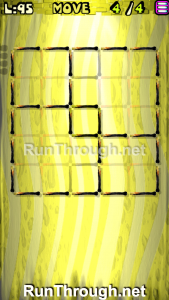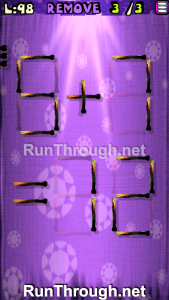# Move the Matches Puzzles Episode 7 Level 81-100 Walkthrough

Walkthrough for all the solutions and answers to the game Move the Matches Puzzles by K-Lab. Get the app on the Google Play Store to play along if you do not already have it. This is for episode 7, levels 81 through 100.

For the walkthrough images, we will be showing the final image and answer of the Matches Puzzle. This will allow you to see where everything goes, and to know for sure that the puzzle works.

###Instructions: Add 3 matches to correct the equation

Solution: 9×8=72

###Instructions: Move 1 match to correct the equation

Solution: 1+8=9

###Instructions: Move 1 match to correct the equation

Solution: 3+8=11

###Instructions: Move 1 match to correct the equation

Solution: 8+8=16

###Instructions: Add 3 matches to correct the equation

Solution: 4×9=36

###Instructions: Add 3 matches to correct the equation

Solution: 7-4=3

###Instructions: Add 2 matches to correct the equation

Solution: 8-2=6

###Instructions: Remove 3 matches to correct the equation

Solution: 8-1=7

###Instructions: Move 2 matches to correct the equation

Solution: 3+2=5

###Instructions: Move 4 matches to get 4 matches

###Instructions: Move 1 match to correct the equation

Solution: 0x7=0

###Instructions: Add 2 matches to correct the equation

Solution: 1×3=3

###Instructions: Add 2 matches to correct the equation

Solution: 4×9=36

###Instructions: Move 1 matches to correct the equation

Solution: 9/1=9

###Instructions: Move 4 matches to get 6 squares

###Instructions: Move 1 match to correct the equation

Solution: 8+9=17

###Instructions: Move 1 match to correct the equation

Solution: 9×9=81

###Instructions: Remove 3 matches to correct the equation

Solution: 5+7=12

###Instructions: Remove 4 matches to correct the equation

Solution: 2×5=10

### Level 100Instructions: Remove 3 matches to get 5 squares